## Polytope

A convex polytope may be defined as the Convex Hull of a finite set of points (which are always bounded), or as the intersection of a finite set of half-spaces. Explicitly, a-dimensional polytope may be specified as the set of solutions to a system of linear inequalities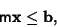where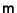is a realMatrix andis a real-Vector. The positions of the vertices given by the above equations may be found using a process called Vertex Enumeration.

A regular polytope is a generalization of the Platonic Solids to an arbitrary Dimension. The Necessary condition for the figure with Schläfli Symbol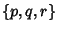to be a finite polytope isSufficiency can be established by consideration of the six figures satisfying this condition. The table below enumerates the six regular polytopes in 4-D (Coxeter 1969, p. 414).

 Name Schläfli Symbol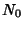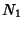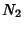Regular Simplex5 10 10 5 Hypercube16 32 24 8 16-Cell8 24 32 16 24-Cell24 96 96 24 120-Cell600 1200 720 120 600-Cell120 720 1200 600

Here,is the number of Vertices,the number of Edges,the number of Faces, andthe number of cells. These quantities satisfy the identitywhich is a version of the Polyhedral Formula.

For-D with, there are only three regular polytopes, the Measure Polytope, Cross Polytope, and regular Simplex (which are analogs of the Cube, Octahedron, and Tetrahedron).

See also 16-Cell, 24-Cell, 120-Cell, 600-Cell, Cross Polytope, Edge (Polytope), Face, Facet, Hypercube, Incidence Matrix, Measure Polytope, Ridge, Simplex, Tesseract, Vertex (Polyhedron)

References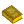Solid Geometry

Coxeter, H. S. M. Regular and Semi-Regular Polytopes I.'' Math. Z. 46, 380-407, 1940.

Coxeter, H. S. M. Introduction to Geometry, 2nd ed. New York: Wiley, 1969.

Eppstein, D. Polyhedra and Polytopes.'' http://www.ics.uci.edu/~eppstein/junkyard/polytope.html.